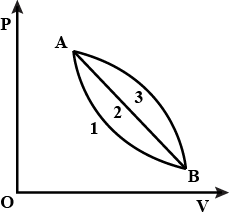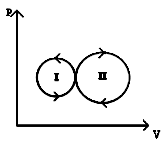A system is taken from state A to state B along two different paths 1 and 2. If the heat absorbed and work done by the system along these two paths are  respectively, then

(1) ${Q}_{1}={Q}_{2}$

(2) ${W}_{1}={W}_{2}$

(3) ${Q}_{1}-{W}_{1}={Q}_{2}-{W}_{2}$

(4) ${Q}_{1}+{W}_{1}={Q}_{2}+{W}_{2}$

Subtopic:  First Law of Thermodynamics |
To view explanation, please take trial in the course below.
NEET 2022 - Target Batch - Aryan Raj Singh
To view explanation, please take trial in the course below.
NEET 2022 - Target Batch - Aryan Raj Singh

Difficulty Level:

In a given process dW = 0, dQ < 0, then for the gas:

1.  Temperature -  increases

2.  Volume - decreases

3.  Pressure - decreases

4.  Pressure - increases

Subtopic:  First Law of Thermodynamics |
To view explanation, please take trial in the course below.
NEET 2022 - Target Batch - Aryan Raj Singh
To view explanation, please take trial in the course below.
NEET 2022 - Target Batch - Aryan Raj Singh

Difficulty Level:

A given mass of gas expands from state A to state B by three paths 1, 2 and 3 as shown in the figure. If respectively be the work done by the gas along the three paths, then:1.

2.

3.

4.

Subtopic:  Work Done by a Gas |
To view explanation, please take trial in the course below.
NEET 2022 - Target Batch - Aryan Raj Singh
To view explanation, please take trial in the course below.
NEET 2022 - Target Batch - Aryan Raj Singh

Difficulty Level:

The ratio of the relative rise in pressure for adiabatic compression to that for isothermal compression is

(1) $\gamma$

(2) $\frac{1}{\gamma }$

(3) 1-$\gamma$

(4) $\frac{1}{1-\gamma }$

Subtopic:  Types of Processes |
To view explanation, please take trial in the course below.
NEET 2022 - Target Batch - Aryan Raj Singh

Difficulty Level:

A sink, that is the system where heat is rejected, is essential for the conversion of heat into work. From which law the above inference follows?

(1) zeroth

(2) First

(3) Second

(4) Third

Subtopic:  Second Law of Thermodynamics |
To view explanation, please take trial in the course below.
NEET 2022 - Target Batch - Aryan Raj Singh
To view explanation, please take trial in the course below.
NEET 2022 - Target Batch - Aryan Raj Singh

Difficulty Level:

For the indicator diagram given below, select the wrong statement:1. Cycle - II is a heat engine cycle.

2. Net work is done on the gas in cycle I.

3. Work done is positive for cycle I.

4. Work done is positive for cycle II.

Subtopic:  Cyclic Process |
To view explanation, please take trial in the course below.
NEET 2022 - Target Batch - Aryan Raj Singh
To view explanation, please take trial in the course below.
NEET 2022 - Target Batch - Aryan Raj Singh

Difficulty Level:

An ideal gas with adiabatic exponent y is heated at constant pressure and it absorbs Q heat. What fraction of this heat is used to perform external work?

1.  $\mathrm{\gamma }$

2.  $\frac{1}{\mathrm{\gamma }}$

3.

4.

Subtopic:  First Law of Thermodynamics |
To view explanation, please take trial in the course below.
NEET 2022 - Target Batch - Aryan Raj Singh
To view explanation, please take trial in the course below.
NEET 2022 - Target Batch - Aryan Raj Singh

Difficulty Level:

Temperature is defined by

1.  First Law of thermodynamics

2.  Second Law of thermodynamics

3.  Third Law of  thermodynamics

4.  Zeroth Law of  thermodynamics

Subtopic:  Basic Terms |
To view explanation, please take trial in the course below.
NEET 2022 - Target Batch - Aryan Raj Singh
To view explanation, please take trial in the course below.
NEET 2022 - Target Batch - Aryan Raj Singh

Difficulty Level:

If 32 gm of ${O}_{2}$ at $27°\mathrm{C}$ is mixed with 64 gm of ${\mathrm{O}}_{2}$ at $327°\mathrm{C}$ in an adiabatic vessel, then the final temperature of the mixture will be :

1.  $200°\mathrm{C}$

2.  $227°\mathrm{C}$

3.  $314.5°\mathrm{C}$

4.  $235.5°\mathrm{C}$

Subtopic:  Molar Specific Heat |
To view explanation, please take trial in the course below.
NEET 2022 - Target Batch - Aryan Raj Singh
To view explanation, please take trial in the course below.
NEET 2022 - Target Batch - Aryan Raj Singh

Difficulty Level:

If ${W}_{1}$ is the work done in compressing an ideal gas from a given initial state through a certain volume isothermally and ${W}_{2}$ is the work done in compressing the same gas from the same initial state through the same volume adiabatically, then:

(1) ${W}_{1}={W}_{2}$

(2) ${W}_{1}<{W}_{2}$

(3) ${W}_{1}>{W}_{2}$

(4) ${W}_{1}=2{W}_{2}$

Subtopic:  Work Done by a Gas |
To view explanation, please take trial in the course below.
NEET 2022 - Target Batch - Aryan Raj Singh
To view explanation, please take trial in the course below.
NEET 2022 - Target Batch - Aryan Raj Singh

Difficulty Level: